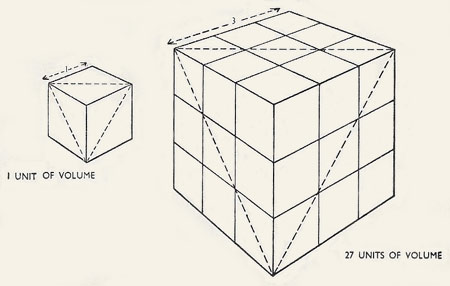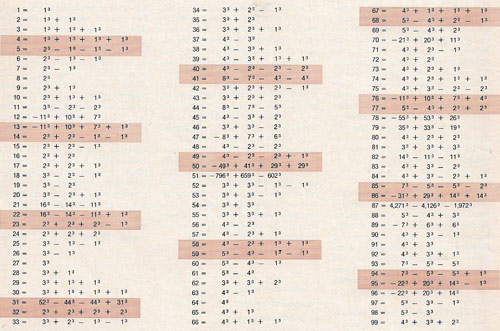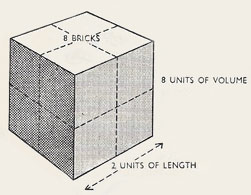A# cubeFig 1. Borg cube from Star Trek.Fig 2. Three cubed equals a volume of 27.Fig 3. Simplest cube expressions known for numbers 1 through 99.

A cube is the Platonic solid that has a square for every one of its six faces; it also has 12 edges and eight vertices (corners). The 60' × 30' × 30' Double Cube Room of Wilton House (the seat of the Earl of Pembroke), near Salisbury, is considered, together with the Single Cube Room of the same domicile, among the finest surviving rooms in England from the mid-seveneenth century. A favorite with film-makers, it has provided locations for Barry Lyndon by Stanley Kubrick, The Madness of King George, and Sense and Sensibility.

A unit cube is a cube with edge length 1.A cube with a side length of two has a volume of eight.A cube with a side length of three has a volume of 27 (Fig 2).

To cube something is to raise it to the power of three. The result of cubing is a cube number: 13 = 1, 23 = 8, 33 = 27, and so on. To take the cube root is the reverse process; thus, 4 cubed (43) is 64 and the cube root of 64 is 4.

A cuboid, also called a rectangular prism, is a hexahedron all of the faces of which are rectangles and all of the opposite faces of which identical.

For cube dissection problems, see Hadwiger problem, Slothouber-Graatsma puzzle, and Soma cube.

## Hypercube

A hypercube is a higher dimensional analog of a cube. A four-dimensional hypercube is known as a tesseract.

## Polycube

A polycube is a polyhedron formed by joining unit cubes by their faces. Examples of puzzles that involve polycubes are the Soma cube and Rubik's cube.